, 郭俊杰1, 吕玉红2
1. 清华大学 机械工程系, 北京 100084;
2. 中国航发沈阳发动机研究所, 沈阳 110015

Nonlinear dynamic characteristics of bolted joints for various pretension forces
, GUO Junjie1, LÜ Yuhong2
1. Department of Mechanical Engineering, Tsinghua University, Beijing 100084, China;
2. AECC Shenyang Engine Research Institute, Shenyang 110015, China
Abstract: The influence of the bolt pretension force on the dynamic characteristics of bolted joints was studied using a double bolt joint with an equivalent single DOF model based on the lumped parameter method near the first mode. Then, the equivalent dynamic parameters were obtained for various bolt preloadings using modal and excitation tests. The equivalent nonlinear dynamic parameters for the joint were then identified using the force-state mapping method for various pretension forces. The results show that the joint nonlinearity increases with decreasing pretension force. Therefore, the nonlinear joint dynamics must be considered for low pretension forces.
Key words: bolted joints     pretension force     dynamic characteristics     parameter identification

1 建立模型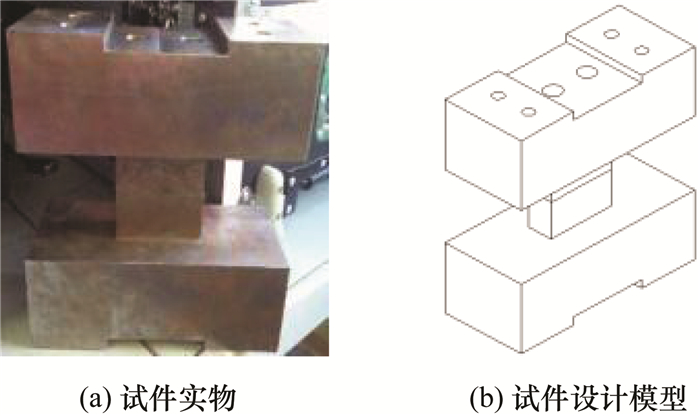图 1 双螺栓结合部试件

 模态阶数 1 2 3 4 5 6 频率/Hz 547.9 848.2 1 081 2 277 2 329 2 860 振型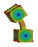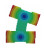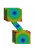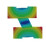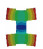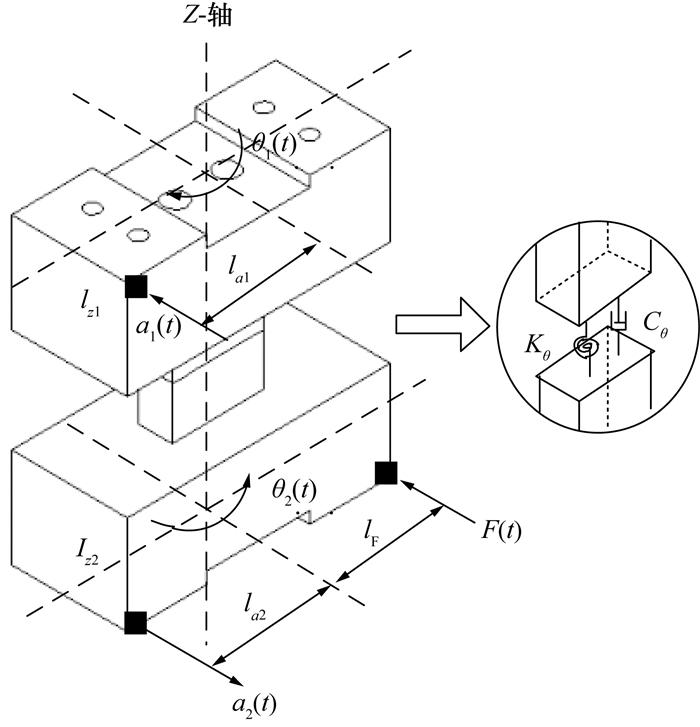图 2 简化的单自由度振动系统

 ${I_{z1}}{{\ddot \theta }_1} = {C_\theta }\left( {{{\dot \theta }_2} - {{\dot \theta }_1}} \right) + {K_\theta }\left( {{\theta _2} - {\theta _1}} \right),$ (1)
 ${I_{z2}}{{\ddot \theta }_2} = {C_\theta }\left( {{{\dot \theta }_1} - {{\dot \theta }_2}} \right) + {K_\theta }\left( {{\theta _1} - {\theta _2}} \right) + {M_z}\left( t \right).$ (2)

 ${I_{z1}} = {I_{z2}} = {I_z},$ (3)

 $\theta = {\theta _2} - {\theta _1}.$ (4)

 ${I_z}\ddot \theta + 2{C_\theta }\dot \theta + 2{K_\theta }\theta = {M_z}\left( t \right).$ (5)

 ${M_z}\left( t \right) = F\left( t \right) \times {l_F},$ (6)
 $\ddot \theta \left( t \right) = {{\ddot \theta }_2} - {{\ddot \theta }_1} \approx \frac{{{a_2}\left( t \right)}}{{{l_{a2}}}} - \frac{{{a_1}\left( t \right)}}{{{l_{a1}}}}.$ (7)

 ${K_{\theta eq}} = 2{K_\theta },$ (8)
 ${C_{\theta eq}} = 2{C_\theta }.$ (9)

 ${I_z}\ddot \theta + {C_{\theta eq}}\dot \theta + {K_{\theta eq}}\theta = {M_z}\left( t \right).$ (10)

 $f = \frac{{{\omega _d}}}{{2{\rm{ \mathsf{ π} }}}} = \frac{{{\omega _n}}}{{2{\rm{ \mathsf{ π} }}}}\sqrt {1 - {\zeta ^2}} .$ (11)

 ${\omega _n} = \sqrt {\frac{{{K_{\theta eq}}}}{{{I_z}}}} ,$ (12)
 $\zeta = \frac{{{C_{\theta eq}}}}{{2{\omega _n}{I_z}}}.$ (13)

ζf通过模态分析得到，由上两式即可计算得到系统一阶模态的等效阻尼和刚度系数CθeqKθeq

 ${I_z}\ddot \theta + {C_{\theta eq}}\dot \theta + {K_{\theta eq}}\theta + f\left( {\dot \theta ,\theta } \right) = {M_z}\left( t \right).$ (14)
2 实验结果 2.1 模态实验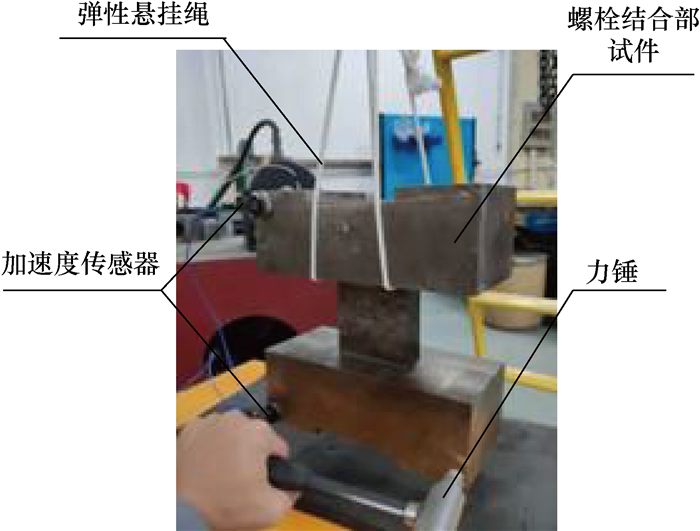图 3 模态测试系统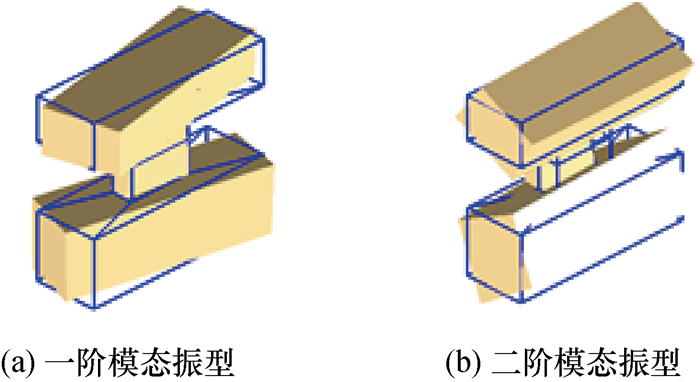图 4 测试模态振型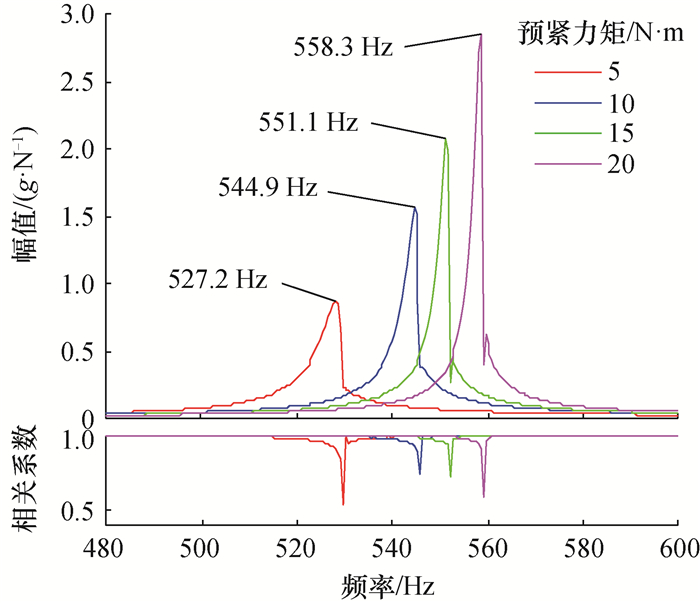图 5 (网络版彩图)一阶模态附近锤击频响函数随预紧力变化

 $\frac{{预紧力矩}}{{\rm N·m}}$ $\frac{{f}}{{\rm Hz}}$ $\frac{{ζ}}{{\%}}$ $\frac{{K_{θeq}}}{{\rm N·m·rad^{-1}}}$ $\frac{{C_{θeq}}}{{\rm N·m·s·rad^{-1}}}$ (5, 5) 527.2 0.5 855 889 33.125 (5, 10) 538.2 0.44 892 006 29.759 (5, 15) 543.5 0.08 909 674 5.464 (5, 20) 547.7 0.06 923 625 4.129 (10, 5) 534.2 0.1 878 669 6.713 (10, 10) 544.9 0.1 914 182 6.847 (10, 15) 551.0 0.07 934 782 4.847 (10, 20) 553.4 0.05 942 991 3.477 (15, 5) 534.8 0.19 880 844 12.770 (15, 10) 546.9 0.07 921 174 4.811 (15, 15) 551.1 0.08 935 088 5.540 (15, 20) 556.0 0.05 951 801 3.493 (20, 5) 537.0 0.09 888 070 6.074 (20, 10) 547.8 0.09 924 027 6.195 (20, 15) 552.9 0.06 941 186 4.168 (20, 20) 558.3 0.05 959 892 3.508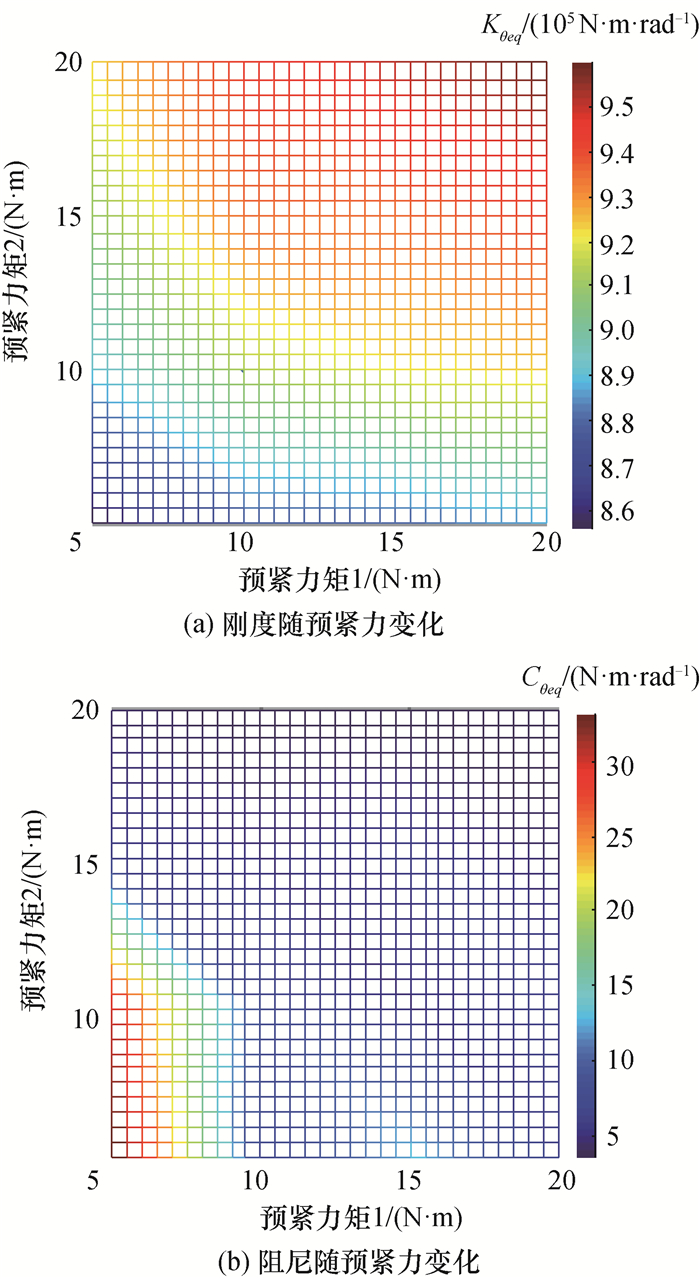图 6 (网络版彩图)模态参数随预紧力变化

2.2 激振实验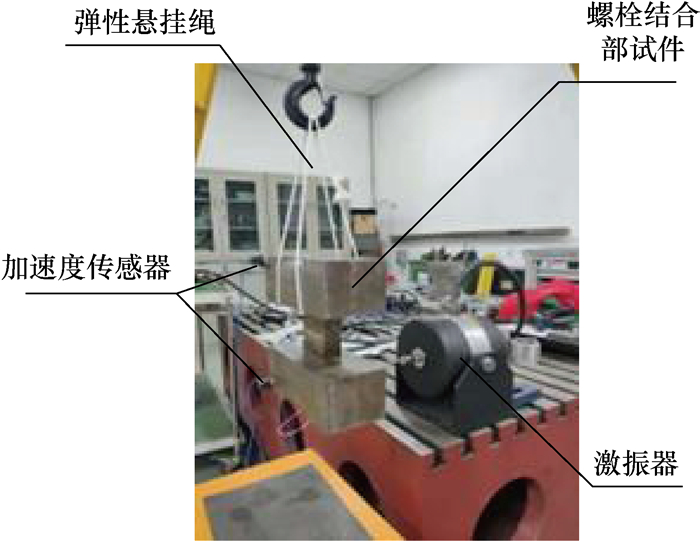图 7 激振测试系统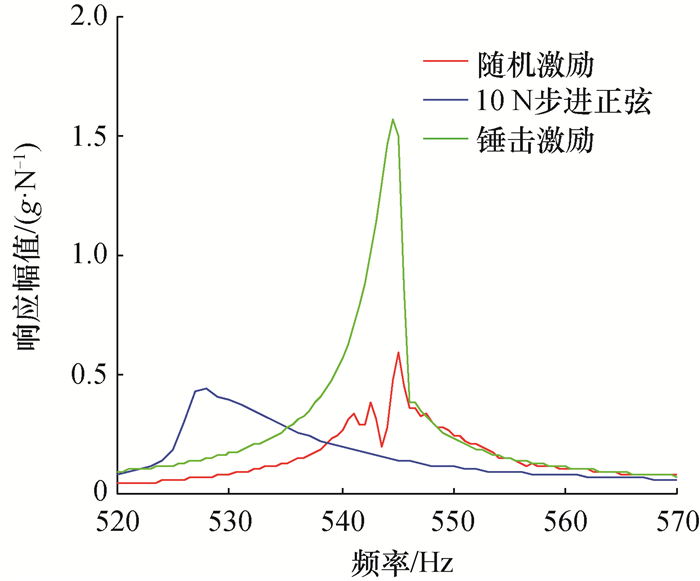图 8 (网络版彩图)不同激励下得到的频响函数对比图 9 加速度响应幅值随力幅变化

3 基于力状态映射法的参数辨识

 ${M_z}\left( t \right) - {I_z}\ddot \theta = {C_{\theta eq}}\dot \theta + {K_{\theta eq}}\theta + f\left( {\dot \theta ,\theta } \right).$ (15)

 $\ddot x = \sum\limits_{i = 1}^n {{a_i}\cos \left( {{\omega _i}t + {\varphi _i}} \right)} ,$ (16)
 $\dot x = \int {\ddot x{\rm{d}}t} = \sum\limits_{i = 1}^n {\frac{{{a_i}}}{{{\omega _i}}}\cos \left( {{\omega _i}t + {\varphi _i} - \frac{{\rm{ \mathsf{ π} }}}{2}} \right)} ,$ (17)
 $x = \int {\dot x{\rm{d}}t} = \sum\limits_{i = 1}^n {\frac{{{a_i}}}{{\omega _i^2}}\cos \left( {{\omega _i}t + {\varphi _i} - {\rm{ \mathsf{ π} }}} \right)} .$ (18)

 $\begin{array}{*{20}{c}} {f\left( {\dot \theta ,\theta } \right) = {a_0} + {a_{01}}\dot \theta + {a_{10}}\theta + {a_{20}}{\theta ^2} + }\\ {{a_{11}}\theta \dot \theta + {a_{02}}{{\left( {\dot \theta } \right)}^2} + {a_{30}}{\theta ^3} + {a_{21}}{\theta ^2}\dot \theta + }\\ {{a_{12}}\theta {{\left( {\dot \theta } \right)}^2} + {a_{03}}{{\left( {\dot \theta } \right)}^3} + {b_1}{\rm{sign}}\left( {\dot \theta } \right).} \end{array}$ (19)

 参数 5 N·m 贡献因子 10 N·m 贡献因子 15 N·m 贡献因子 20 N·m 贡献因子 a0 0.012 0.070 0.023 0.095 0.037 0.112 0.059 0.160 a01 9.548 3.705 5.905 2.214 5.159 1.894 4.695 1.694 a10 780 372 95.445 865 020 97.017 908 750 97.609 937 372 97.707 a02 -2.655 0.086 -2.798 0.116 -2.515 0.137 -3.326 0.196 a11 637.409 0.004 -408.134 0.003 1 495.226 0.014 199.938 0.002 a03 76.706 0.216 40.275 0.195 -0.156 0.001 13.640 0.138 a12 -926 782 0.369 -388 915 0.253 -114 917 0.128 -34 588 0.045 b1 -0.018 0.106 -0.026 0.107 -0.035 0.106 -0.022 0.059 注:未列出项贡献因子小于0.001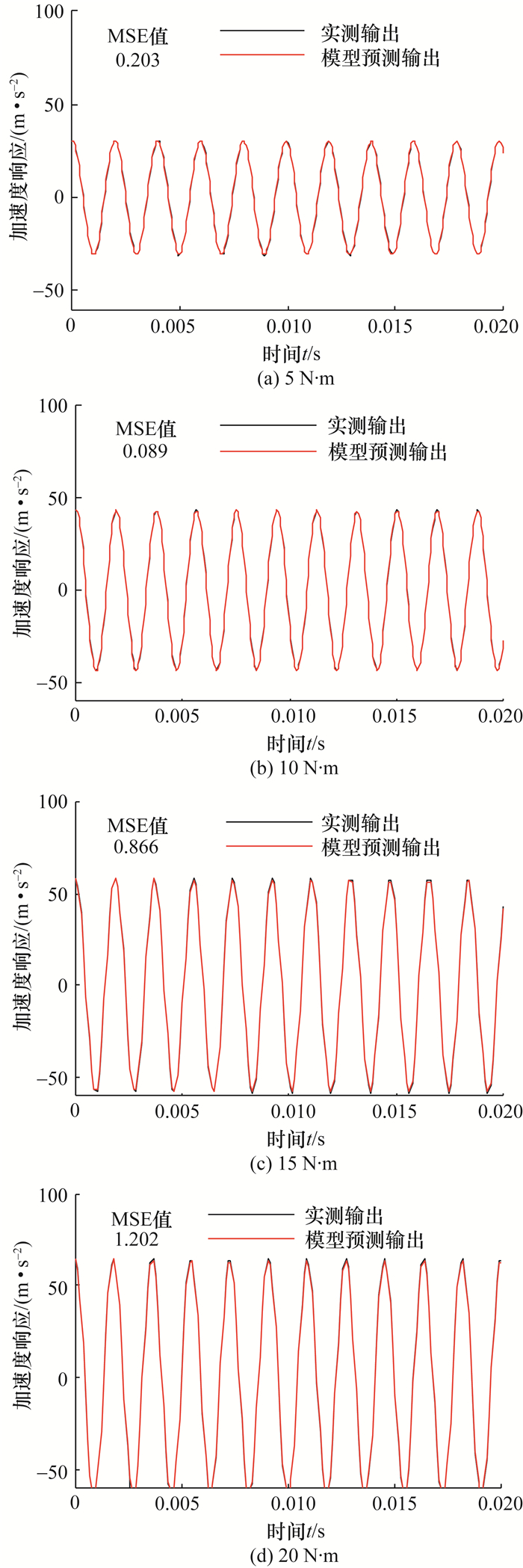图 10 (网络版彩图)仿真结果与实验结果对比

 等效参数 测试条件 5 N·m 10 N·m 15 N·m 20 N·m 刚度/(105 N·rad-1) 模态测试 8.56 9.14 9.35 9.60 正弦激励 7.80 8.65 9.09 9.37 误差/% 8.82 5.38 2.82 2.35 阻尼比/% 模态测试 0.50 0.10 0.08 0.05 正弦激励 0.152 0.089 0.076 0.068 误差/% 69.7 10.9 5.10 36.1

4 结论

1) 螺栓预紧力对于结合部动态特性有重要影响，锤击模态刚度随预紧力降低而降低，模态阻尼比随预紧力降低而增加，并且预紧力较低时变化更加显著。

2) 对于同一低阶模态，结合面有多个螺栓作用时，每个螺栓预紧力对于模态参数变化的影响趋势相同，因此同一模态频率或阻尼比可能对应多组预紧力值。

3) 螺栓结合部存在一定程度的刚度渐软非线性，结合部的等效动力学参数会随着激励信号变化而变化，并且预紧力越低，结合部非线性程度越强，等效动力学参数受激励信号的影响越大，因此在预紧力较低时对结合部的准确建模不能忽略非线性因素的影响。

  IBRAHIM R A, PETTIT C L. Uncertainties and dynamic problems of bolted joints and other fasteners[J]. Journal of Sound and Vibration, 2005, 279(3-5): 857-936. DOI:10.1016/j.jsv.2003.11.064  叶佩青, 王仁彻, 赵彤, 等. 机床整机动态特性研究进展[J]. 清华大学学报(自然科学版), 2012, 52(12): 1758-1763. YE P Q, WANG R C, ZHAO T, et al. Recent research advances of whole machine tool dynamics[J]. Journal of Tsinghua University (Science and Technology), 2012, 52(12): 1758-1763. (in Chinese)  蔡力钢, 王峰, 李玲, 等. 栓接结合部动态特性研究进展[J]. 机械工程学报, 2013, 49(9): 158-168. CAI L G, WANG F, LI L, et al. Review on dynamic properties of bolted joints[J]. Journal of Mechanical Engineering, 2013, 49(9): 158-168. (in Chinese)  ČELIČ D, BOLTEŽAR M. Identification of the dynamic properties of joints using frequency-response functions[J]. Journal of Sound and Vibration, 2008, 317(1-2): 158-174.  董冠华, 殷勤, 殷国富, 等. 机床结合部动力学建模与辨识方法的研究[J]. 机械工程学报, 2012, 52(5): 162-168. DONG G H, YIN Q, YIN G F, et al. Research on dynamics modeling and identification of machine tool joints[J]. Journal of Mechanical Engineering, 2012, 52(5): 162-168. (in Chinese)  张辉, 于长亮, 王仁彻, 等. 机床支撑地脚结合部参数辨识方法[J]. 清华大学学报(自然科学版), 2014, 54(6): 815-821. ZHANG H, YU C L, WANG R C, et al. Parameters identification method for machine tool support joints[J]. Journal of Tsinghua University (Science and Technology), 2014, 54(6): 815-821. (in Chinese)  张学良, 范世荣, 温淑花, 等. 基于等效横观各向同性虚拟材料的固定结合部建模方法[J]. 机械工程学报, 2017, 53(15): 141-147. ZHANG X L, FAN S R, WEN S H, et al. Modeling method of fixed joint interfaces based on equivalent transversely isotropic virtual material[J].